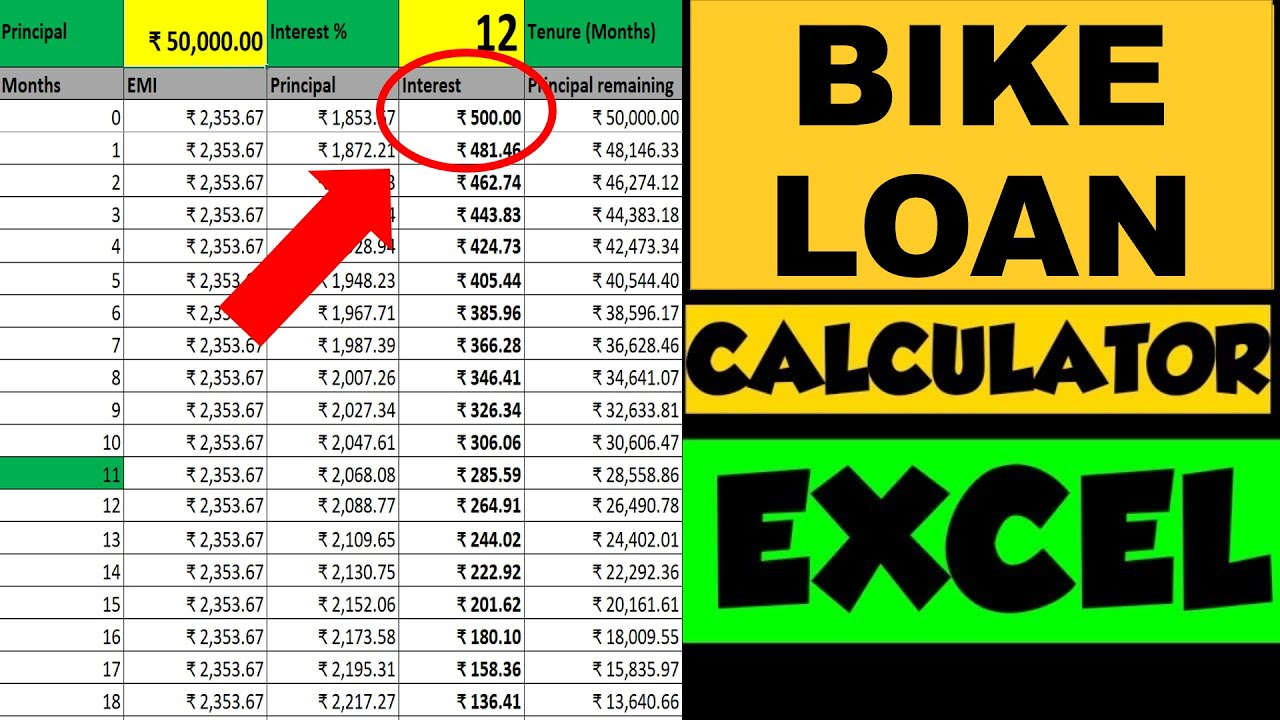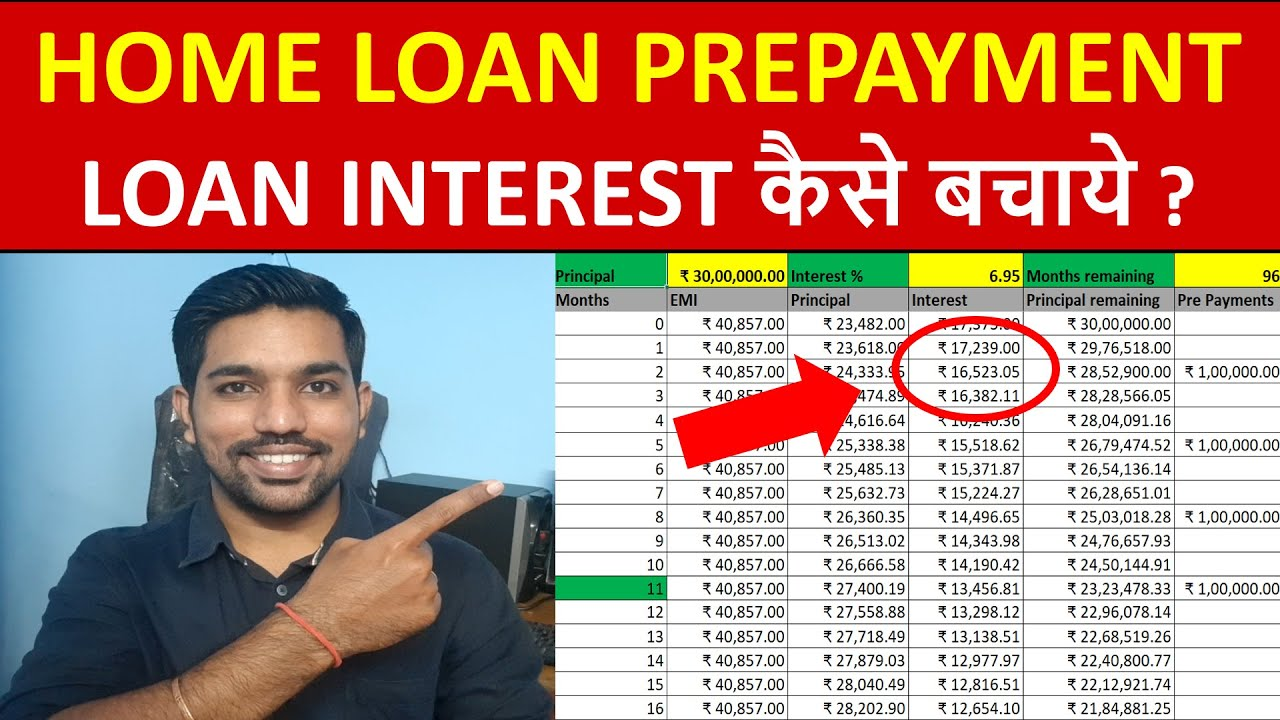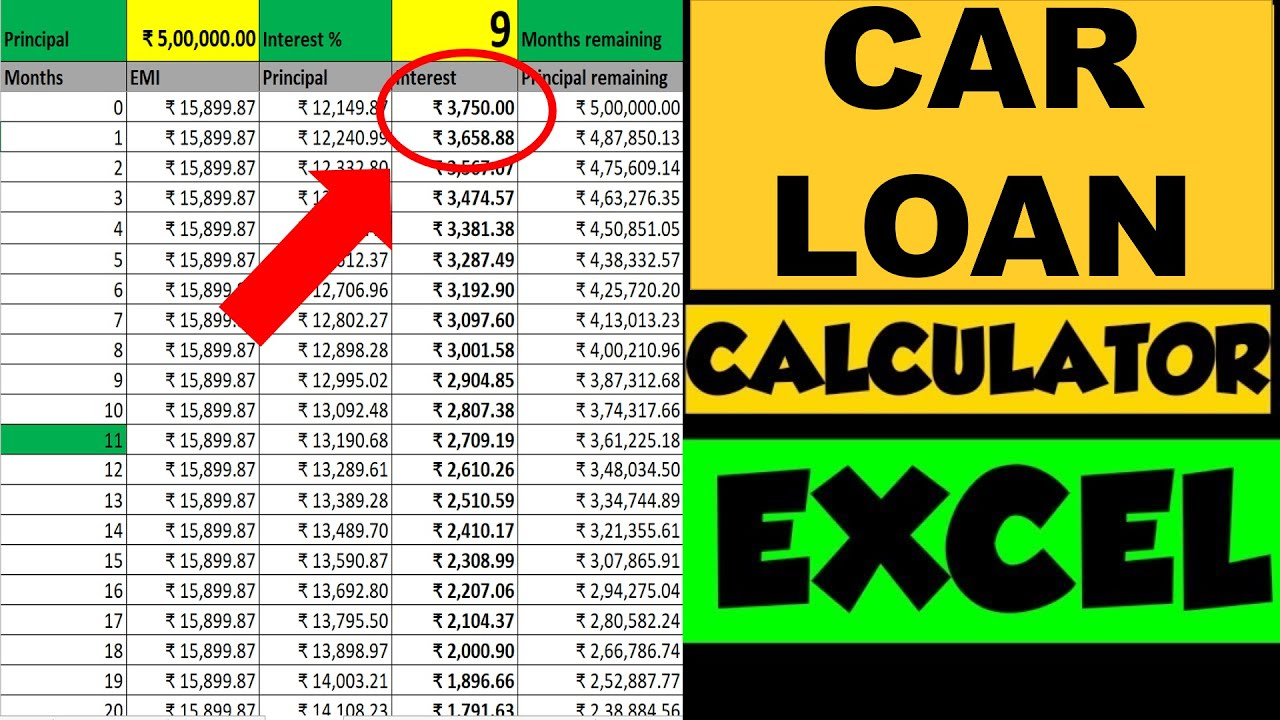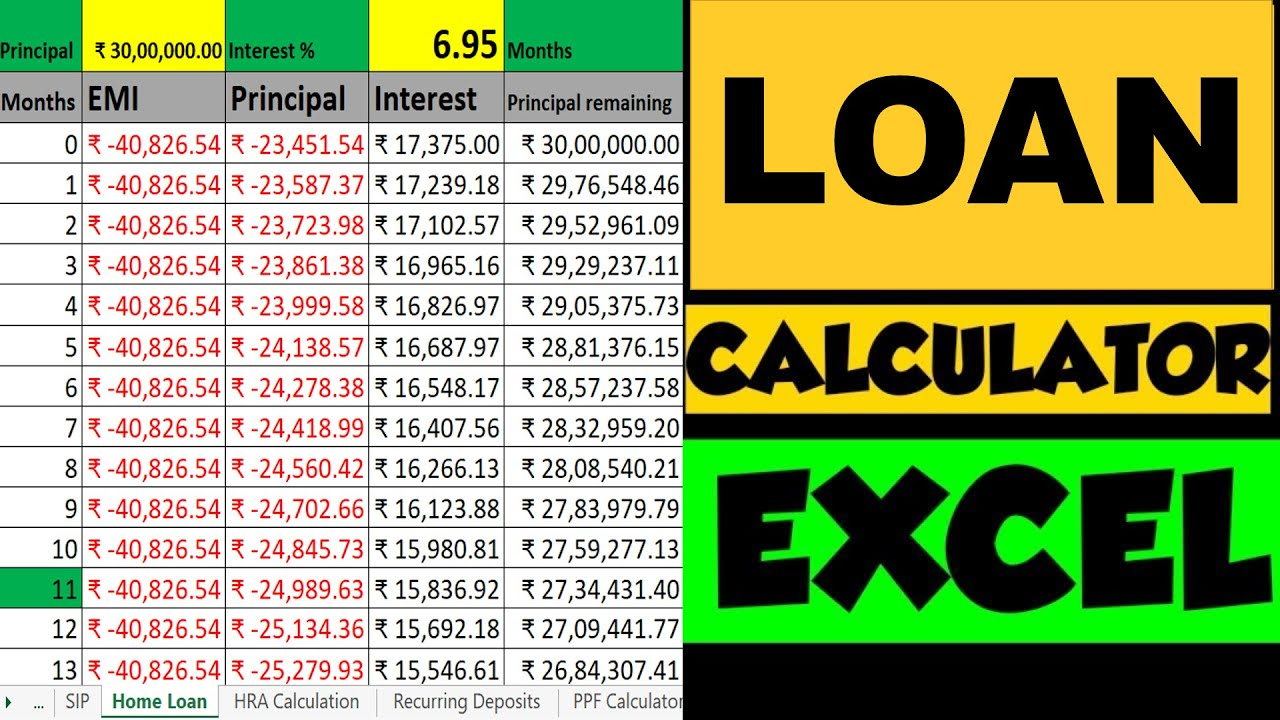# Bike Loan EMI Calculator using Excel | Principal & Interest AmountsOne of the most asked questions among youth of India on my YouTube Channel is – how to calculate Bike Loan EMI in India and how banks use their Bike loan calculator to calculate EMI of the loan for a bike we want to buy? In this article we’ll cover everything you need to know about Bike Loan EMI and also use excel calculator to calculate the principal and interest amounts in Bike Loan.

When we calculate EMI (Equated monthly installment) of any loan, the EMI consists of 2 components – Principal and Interest Amounts. We can easily use the excel function PMT() to calculate EMI of our bike loan. Major financial institutions including banks such as SBI, HDFC, ICICI, bajaj finance, etc. use this same formula to calculate EMI of your bike loan and interest amount that you will be paying. Interest amount is something you pay extra apart from the principal amount that you take as loan which you have to pay. The only variable component here is the total interest amount which varies and depends on the interest rate, tenure and the principal amount of your bike loan.

## Bike Loan EMI Calculator Video:Watch more Excel Videos Here

Watch full video to know how much interest you’ll pay in your Bike loan using examples and how you can decrease interest amount that you have to pay.

## What is Bike Loan EMI

• EMI stands for Equated Monthly Installment
• It includes repayment of the principal amount and payment of the interest on the outstanding amount of your bike loan
• A longer loan tenure helps in reducing the EMI, but increases the interest amount you pay on your bike loan. This increases the total amount you have to pay back to bank or financial institution while repaying your loan amount
• Opt for higher bike loan EMI to reduce your interest amount and tenure. But again, you have to see how much EMI you can afford every month
• Keeping the tenure low also reduces the total interest amount you pay back to bank. This happens since you finish off your loan as early as possible which is a good step in paying less interest amount

More Recommended Books Here

## Things to Know about Bike Loan EMI

• There are 2 parts of your EMI: Principal amount Interest Amount
• Interest Amount is high initially during your Bike Loan tenure
• Principal amount is less initially during your Bike Loan tenure
• High interest amount is already taken at the initial bike loan period from your EMIs
• You should pre pay most of your principal amount if you want to decrease maximum interest amount in your Bike Loan
• You can easily calculate your Bike loan EMI using excel calculator as we can see in above video
• Pre pay your bike loan principal amount initially as much as you can to decrease interest amount that you have to pay throughout your loan tenure
• Increase in bike loan tenure will increase the interest amount you’ll pay throughout your bike loan tenure
• So, keep your tenure and interest rate as low as possible, pay back some additional principal amount in the form of prepayment to reduce your outstanding principal amount to decrease your interest amount you’ll be paying in your bike loan

## Importance of Bike Loan Interest Rate

• It is very important to know the interest rate of loan
• Compare the interest rates from various banks and financial institutions before applying for bike loan
• Know the difference between fixed and floating interest rates
• Go for floating interest rates to get benefits of interest rates decrease
• Your interest amount in every EMI depends on this Interest rate
• More interest rate means more interest amount that you pay on your loan. Less interest rate means less interest amount you pay on your loan.

ALSO READ: Best Mutual Funds to Invest

## How Bike Loan Excel Calculator helps you

• You can provide the bike loan tenure and loan interest rate in order to know how much interest you’ll pay
• You can also check the decrease in principal amount if you pre pay some of the principal amount

## How to Save Bike Loan Interest

• You can easily decrease the bike loan interest amount by prepaying your principal amount
• Bike Loan interest amount is calculated based on your outstanding principal amount remaining
• So in order to decrease the interest that you’ll pay, you need to decrease your outstanding principal amount
• The sooner you decrease your outstanding principal amount at the initial stages of loan tenure, more interest you’ll be saving

You can watch this below video to see how Home Loan Prepayments will help save Home Loan interest using Excel Examples. With Home Loan Prepayment of Rs. 1 Lac, you can save up to Rs. 68,442 in your entire Home Loan Tenure. Watch this video to see the calculation in excel. Similar to home loan prepayments, you can do Bike Loan prepayments as well based on your affordability

### Home Loan Prepayment Calculation VideoWatch more Excel Videos Here

## Bike Loan Calculation Example

Let’s now see how bike loan calculation works with the help of example

Let’s consider your Bike loan of principal amount = Rs. 50,000, tenure = 3 years (36 months) and interest rate = 12%. Providing these inputs in our excel calculator, you get EMI = Rs. 1,660.72 as seen below:

As you notice, principal amount is lowest initially and increases with every month after you pay EMIs. On the other hand, interest amount is highest initially and decreases with time after your EMIs are paid every month.

The total interest you will be paying is Rs. 9,785.76 (on top right) and the total amount will be this interest amount + principal amount you took as bike loan = Rs. 59,785.76 (on top right)

So overall, this excel calculator helps you know your EMI, Principal and interest amounts every month you pay via EMI, total interest you’ll be paying in entire tenure and the total amount you pay back to the bank or financial institution.

ALSO READ: Savings Account Interest Calculation using Excel

## How to Save Bike Loan Interest

As mentioned above, you can make some Bike loan prepayments in order to save your loan interest. Loan prepayment is the method to reduce your principal outstanding balance which you can see above in column E (principal remaining), based on this amount, your every month interest is calculated.

And since you get interest amount using this principal remaining and interest rate, and you already have the EMI amount calculated, the remaining amount becomes your principal amount for that month.

This is why, your principal remaining or outstanding principal amount is deciding factor for the interest amount you’ll pay every month. Reducing this amount gradually as earl as possible (based on your affordability), you can reduce the interest amounts substantially.

Let’s say for above example, you are able to accumulate Rs. 10,000 in 5th month and make prepayment in this month, as seen below you’ll be saving interest amount of Rs. 3,275.71 throughout the loan tenure.

The prepayment amount you paid is subtracted from your principal remaining balance which helped in saving the interest amount throughout the tenure. Similarly you can make multiple prepayments to save more loan interest amounts.

And this is how you will save bike loan interest amount as this is the only amount which is variable. Principal amount will have to be paid in full.

Important: While making prepayment, we assumed to reduce the tenure instead of EMI. When you make prepayment, you have 2 options – to reduce EMI or tenure. You’ll save more interest amount while keeping the EMI same and reducing tenure as seen above.

## Some more Videos